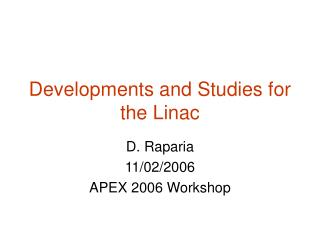DownloadDownload PresentationDevelopments and Studies for the Linac

# Developments and Studies for the Linac

Download Presentation## Developments and Studies for the Linac

- - - - - - - - - - - - - - - - - - - - - - - - - - - E N D - - - - - - - - - - - - - - - - - - - - - - - - - - -
##### Presentation Transcript

1. Developments and Studies for the Linac D. Raparia 11/02/2006 APEX 2006 Workshop

2. Outlines • Energy spread reduction of polarized proton from Linac (AP issue) • Injecting polarized proton at 116 MeV (Reliability & Cost issue)

3. Energy Spread Reduction of Polarized Proton from Linac • 1989 tech note (Brennan,Ahrens,Alessi) dp/p =  0.14% (full max ?) • 1999 Down HEBT (0.5 mA H-) dp/p =  0.16% FWFM  0.076 FWHM • 2000-2004 dp/p =  0.067 to  0.07 FWHM (polarized proton) • Can we reduce it ? • Do we want to reduce it ?

4. Energy Spread as function of T8 & T9 Phases (PARMILA Calculation) E Operating 0  360  Tank 8 Tank 9 0  360 

5. Measurement in HEBT1999 (I=0.5 mA H-) PARMILA p/p=  0.015%(rms) Measurement p/p=  0.080% FWFM p/p=  0.053% FWHM T8 & T9  +180  T=158 MeV =0.517, =1.168 =0.604 2 3=0.426 PARMILA p/p= 0.11%(rms) Measurement p/p=  0.160% FWFM p/p=  0.076% FWHM Normal Operating phase T=200 MeV =0.566, =1.213 0.687 2 3=0.572 Measurement p/p=  0.088% FWFM p/p=  0.053% FWHM T8  = +180 T=162 MeV =0.522, =1.173 =0.612 2 3=0.440

6. Consequences of Lower Energy • Space charge tune spread Pulse length 350s,I=0.5mA=> N=1.1 x 1012; x= y=1.0 cm, coasting beam @200MeV=0.007;@162MeV=0.009;@158MeV=0.0097 • Emittance growth due to foil scattering Foil Thickness 100 g/cm2. ; 350 turn injection LTB: x(n,95%)=6.87, y(n,95%)=13.64; Beta function at injection: x=10.9 m, y=4.9 m 200 MeV 162 MeV 158 MeV rms (mrad) 0.529 0.643 0.658 x(n,95%,  mm mrad) 14.8 18.6 19.1 y(n,95%,  mm mrad) 15.0 15.8 15.9 Effstrip(%) 91.0 94.6 94.9 • Depolarization due to emittance growth (?) • RHIC luminosity may not be effected, we do transverse and longitudinal scrapping in Booster

7. Lower Energy Injection into Booster • BLIP run at 116 MeV with last four tanks off • If we run polarized proton at the 116 MeV: + Linac reliability will increase (9/5) + Linac operation cost will decrease only 5 RF system instead of 9 \$33,705 +\$110,875=\$144,580 for 10 weeks Power Tube - Emittance growth due to higher multiple scattering angle at foil - Depolarization due to higher emittance (?)

8. Space Charge Tune Spread =0.5 N r0 C/(422 3 y(x+ y)) N=1.1 1012, r0=1.54 10-18, C=200m x= y=1.0 cm, =5.2, coasting beam 200 MeV = 0.007, 2 3 = 0.572 116 MeV = 0.014, 2 3 = 0.295

9. Emittance Growth due to Multiple Scattering at foil LTB: x(n,95%)=6.87, y(n,95%)=13.64; Beta function at injection: x=10.9 m, y=4.9 m 200 MeV 116 MeV Ratio  0.566 0.456 1.24  1.213 1.124 1.08  0.687 0.513 1.34 2 3 0.572 0.295 1.94 Number of Turns 290 360 1.24 foil thickness (g/cm2) 100 65 1.54 Effstrip (%)91.0 91.0 1.00 rms (mr) 0.529 0.693 0.78 x(n,95%, mm mrad) 14.8 18.1 0.82 y(n,95%,  mm mrad) 15.0 15.7 0.96 p/p (%) (MWHM) 0.07(Meas) 0.07 (cal) 1.00

10. Conclusions • Energy spread reduction of polarized proton from linac can be reduced but at lower energy • Injecting polarized proton at 116 MeV, linac becomes more reliable (9/5) and one can save about \$145K for 10 week run for power and tube cost